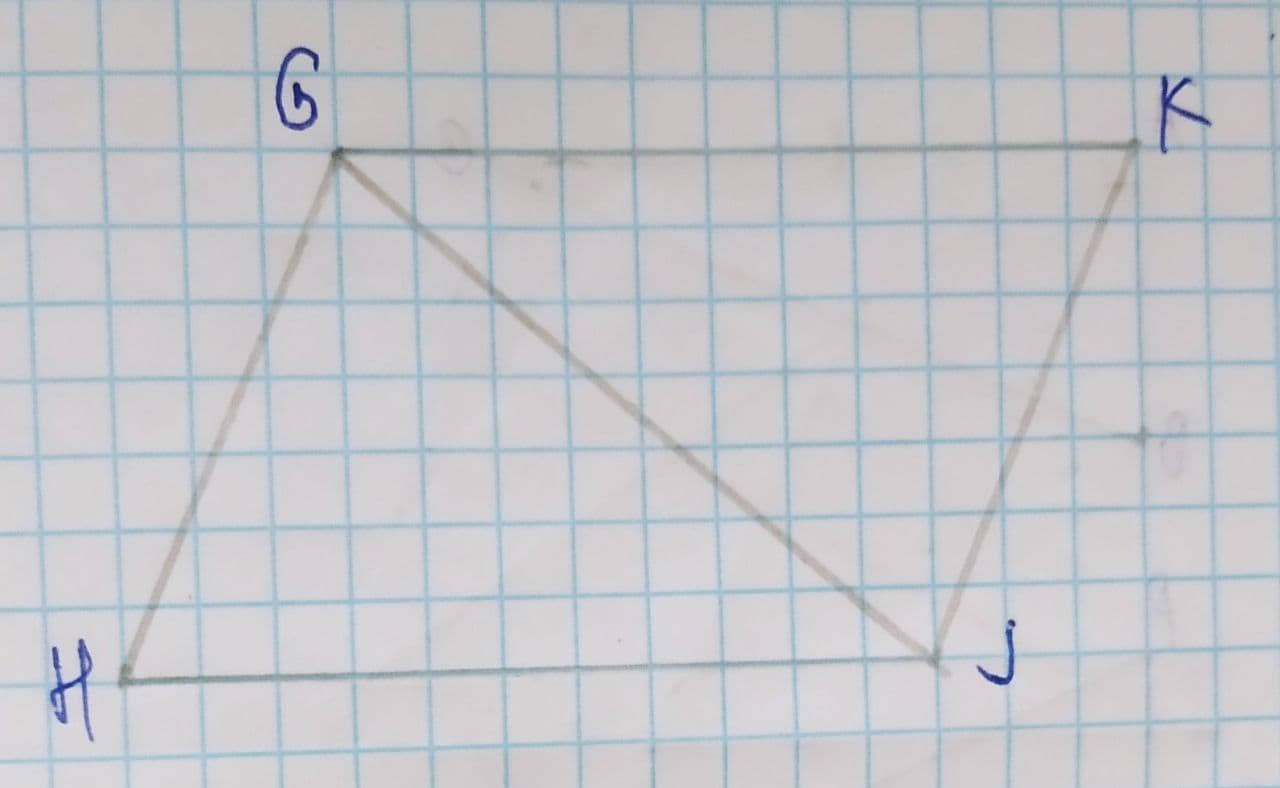Question# \angle HGJ\cong \angle KJG, \angle KGJ\cong \angle HJG show that two sides are congruent.

Congruence
ANSWEREDTo show that two sides are congruent.
Given information:
$$\displaystyle\angle{H}{G}{J}\stackrel{\sim}{=}\angle{K}{J}{G}$$
$$\displaystyle\angle{K}{G}{J}\stackrel{\sim}{=}\angle{H}{J}{G}$$2021-07-27

Formula used:
The below property is used:
Two triangles are congruent by ASA congruence rule.
Proof:
From figure, we getIt is given that,
$$\displaystyle\angle{H}{G}{J}\stackrel{\sim}{=}\angle{K}{J}{G}$$
$$\displaystyle\angle{K}{G}{J}\stackrel{\sim}{=}\angle{H}{J}{G}$$
By reflexive property, we get
$$\displaystyle\overline{{{G}{J}}}\stackrel{\sim}{=}\overline{{{G}{J}}}$$
By ASA congruence rule, we get
$$\displaystyle\triangle{G}{H}{J}\stackrel{\sim}{=}\triangle{J}{K}{G}$$
As corresponding parts of congruent triangles are congruent, we get
$$\displaystyle\overline{{{H}{G}}}\stackrel{\sim}{=}\overline{{{K}{J}}}$$